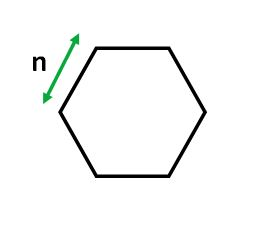# Area of a Hexagon

A hexagon is a 6-sided, 2-dimensional geometric figure. The total of the internal angles of any hexagon is 720°. A regular hexagon has 6 rotational symmetries and 6 reflection symmetries. All internal angles are 120 degrees.Examples :

```Input: 4
Output: 41.5692

Input: 6
Output: 93.5307```

## Recommended: Please try your approach on {IDE} first, before moving on to the solution.

Number of vertices: 6
Number of edges: 6
Internal angle: 120°
Area = (3 √3(n)2 ) / 2

How does the formula work? There are mainly 6 equilateral triangles of side n and area of an equilateral triangle is (sqrt(3)/4) * n * n. Since in hexagon, there are total 6 equilateral triangles with side n, are of the hexagon becomes (3*sqrt(3)/2) * n * n

## C++

 `// CPP program to find  ` `// area of a Hexagon ` `#include ` `#include ` `using` `namespace` `std; ` ` `  `// function for calculating ` `// area of the hexagon. ` `double` `hexagonArea(``double` `s) ` `{ ` `    ``return` `((3 * ``sqrt``(3) *  ` `            ``(s * s)) / 2);      ` `} ` ` `  `// Driver Code ` `int` `main() ` `{ ` `    ``// Length of a side  ` `    ``double` `s = 4;  ` `    ``cout << ``"Area : "` `         ``<< hexagonArea(s); ` `    ``return` `0; ` `} `

## Java

 `class` `GFG  ` `{  ` `    ``// Create a function for calculating ` `    ``// the area of the hexagon. ` `    ``public` `static` `double` `hexagonArea(``double` `s)  ` `    ``{ ` `        ``return` `((``3` `* Math.sqrt(``3``) *  ` `                ``(s * s)) / ``2``); ` `    ``}  ` `         `  `    ``// Driver Code ` `    ``public` `static` `void` `main(String[] args)  ` `    ``{      ` `        ``// Length of a side ` `        ``double` `s = ``4``;       ` `        ``System.out.print(``"Area: "` `+  ` `                          ``hexagonArea(s) ); ` `    ``} ` `} `

## Python3

 `# Python3 program to find ` `# area of a Hexagon ` `import` `math ` ` `  `# Function for calculating  ` `# area of the hexagon. ` `def` `hexagonArea(s): ` `     `  `    ``return` `((``3` `*` `math.sqrt(``3``) ``*`  `            ``(s ``*` `s)) ``/` `2``);  ` `     `  `# Driver code      ` `if` `__name__ ``=``=` `"__main__"` `:  ` ` `  `    ``# length of a side.  ` `    ``s ``=` `4` ` `  `    ``print``(``"Area:"``,``"{0:.4f}"` `.  ` `           ``format``(hexagonArea(s))) ` ` `  `# This code is contributed by Naman_Garg `

## C#

 `// C# program to find ` `// area of a Hexagon ` `using` `System; ` ` `  `class` `GFG  ` `{ ` `     `  `    ``// Create a function for calculating ` `    ``// the area of the hexagon. ` `    ``public` `static` `double` `hexagonArea(``double` `s)  ` `    ``{ ` `        ``return` `((3 * Math.Sqrt(3) *  ` `                ``(s * s)) / 2); ` `    ``}  ` `         `  `    ``// Driver Code ` `    ``public` `static` `void` `Main()  ` `    ``{ ` `        ``// Length of a side  ` `        ``double` `s = 4;  ` `         `  `        ``Console.WriteLine(``"Area: "` `+  ` `                           ``hexagonArea(s) ); ` `    ``} ` `} ` ` `  `// This code is contributed by vt_m. `

## PHP

 ` `

Output :

`Area: 41.5692`

Attention reader! Don’t stop learning now. Get hold of all the important DSA concepts with the DSA Self Paced Course at a student-friendly price and become industry ready.

My Personal Notes arrow_drop_upCheck out this Author's contributed articles.

If you like GeeksforGeeks and would like to contribute, you can also write an article using contribute.geeksforgeeks.org or mail your article to contribute@geeksforgeeks.org. See your article appearing on the GeeksforGeeks main page and help other Geeks.

Please Improve this article if you find anything incorrect by clicking on the "Improve Article" button below.

Improved By : vt_m, jit_t, Naman_Garg